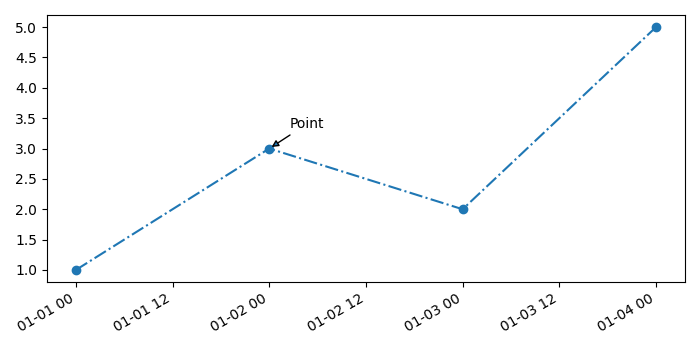# Annotate Time Series plot in Matplotlib

To annotate time series plot in matplotlib, we can take the following steps −

• Create lists for time and numbers.

• Using subplots() method, create a figure and a set of subplots.

• Using plot_date() method, plot the data that contains dates with linestyle "-.".

• Annotate a point in the plot using annotate() method.

• Date ticklabels often overlap, so it is useful to rotate them and right-align them.

• To display the figure, use show() method.

## Example

import datetime as dt
from matplotlib import pyplot as plt, dates as mdates
plt.rcParams["figure.figsize"] = [7.00, 3.50]
plt.rcParams["figure.autolayout"] = True
x = [dt.datetime(2021, 1, 1), dt.datetime(2021, 1, 2),
dt.datetime(2021, 1, 3), dt.datetime(2021, 1, 4)]
y = [1, 3, 2, 5]
fig, ax = plt.subplots()
ax.plot_date(x, y, linestyle='-.')
ax.annotate('Point', (mdates.date2num(x), y), xytext=(15, 15),
textcoords='offset points', arrowprops=dict(arrowstyle='-|>'))
fig.autofmt_xdate()
plt.show()

## Output